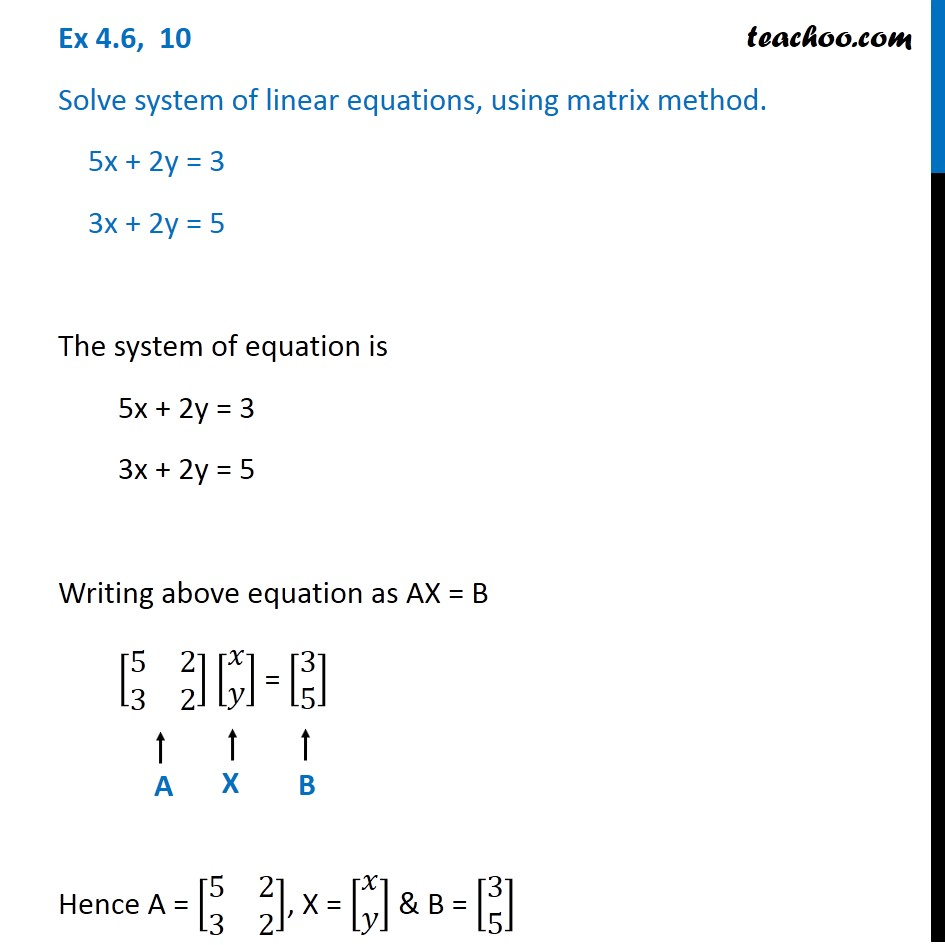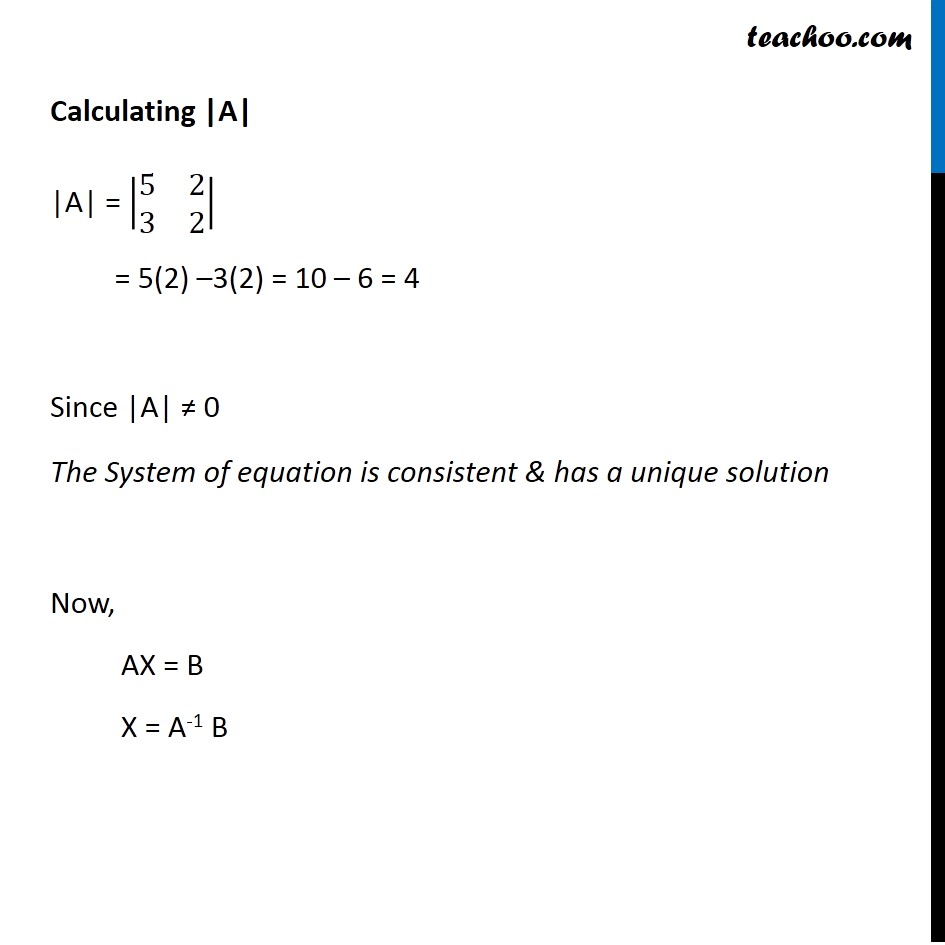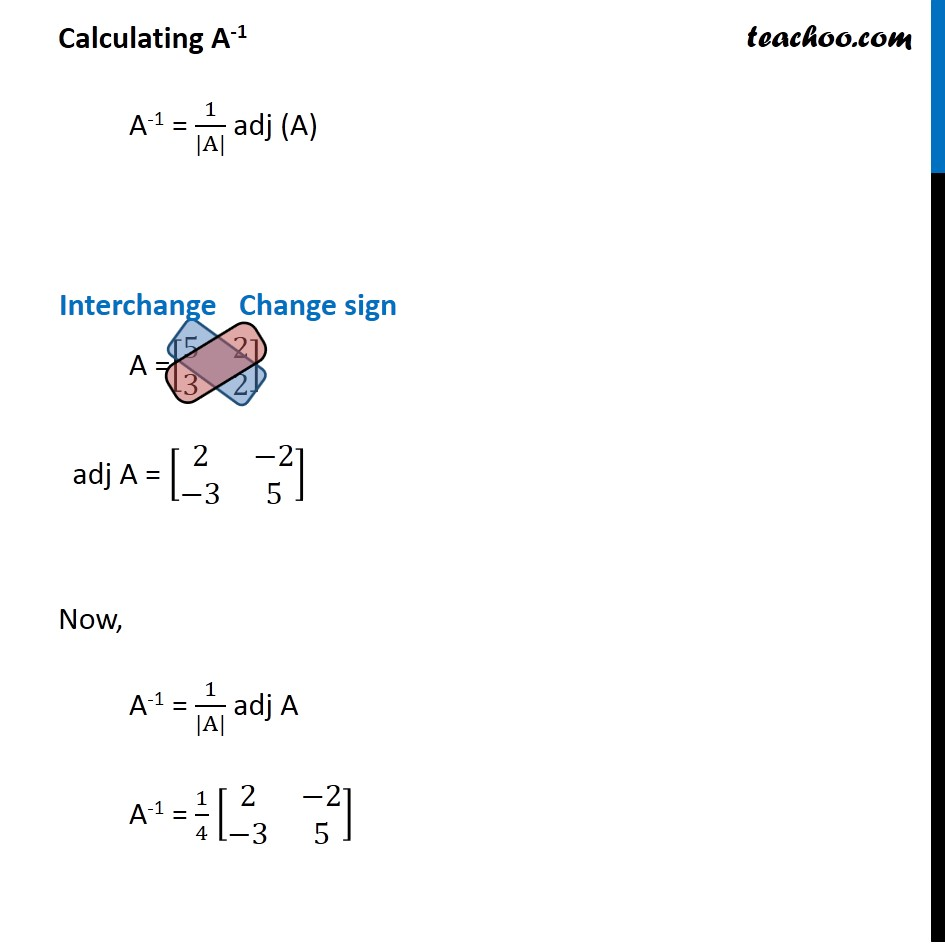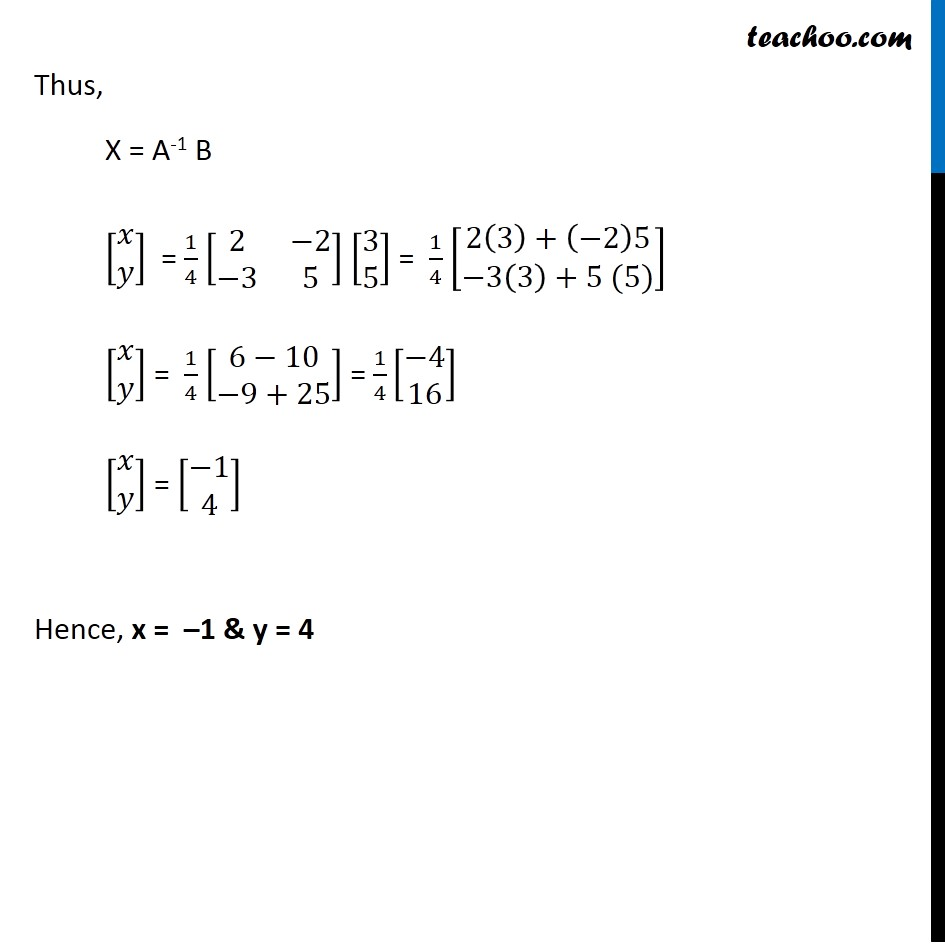Ex 4.6

Chapter 4 Class 12 Determinants
Serial order wiseGet live Maths 1-on-1 Classs - Class 6 to 12

### Transcript

Ex 4.6, 10 Solve system of linear equations, using matrix method. 5x + 2y = 3 3x + 2y = 5 The system of equation is 5x + 2y = 3 3x + 2y = 5 Writing above equation as AX = B [■8(5&[email protected]&2)] [■8(𝑥@𝑦)] = [■8([email protected])] Hence A = [■8(5&[email protected]&2)], X = [■8(𝑥@𝑦)] & B = [■8([email protected])] Calculating |A| |A| = |■8(5&[email protected]&2)| = 5(2) –3(2) = 10 – 6 = 4 Since |A| ≠ 0 The System of equation is consistent & has a unique solution Now, AX = B X = A-1 B Calculating A-1 A-1 = 1/(|A|) adj (A) A =[■8(5&[email protected]&2)] adj A = [■8(2&−[email protected]−3&5)] Now, A-1 = 1/(|A|) adj A A-1 = 1/4 [■8(2&−[email protected]−3&5)] Thus, X = A-1 B [■8(𝑥@𝑦)] = 1/4 [■8(2&−[email protected]−3&5)] [■8([email protected])] = 1/4 [■8(2(3)+(⤶7−2)[email protected]−3(3)+5 (5))] [■8(𝑥@𝑦)] = 1/4 [■8(6−[email protected]−9+25)] = 1/4 [■8(−[email protected])] [■8(𝑥@𝑦)] = [■8(−[email protected])] Hence, x = –1 & y = 4